Lec 3 - Trigonometric Integrals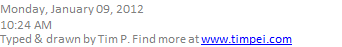Recall: Techniques of Integration

1. Substitution
1. Integration by Parts

(7.2)

Example

Find a reduction formula:Solution: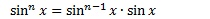Use integration by parts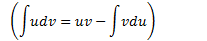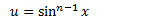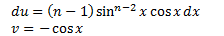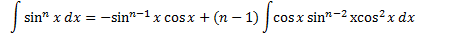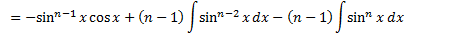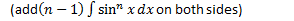Example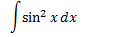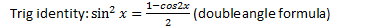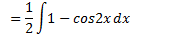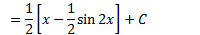Example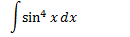(could apply reduction formula or half angle formula)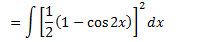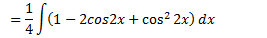(use double angle formula again)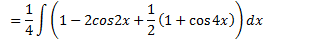Now we consider odd powers.

Example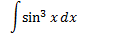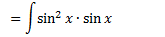(use trig identity)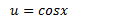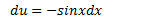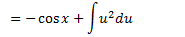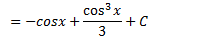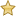Generally,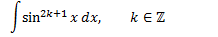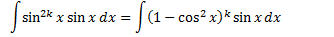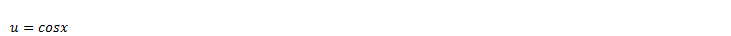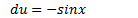You expand the polynomial and then integrate each term.More generally, consider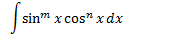There are 3 cases to consider.

1.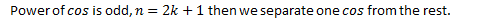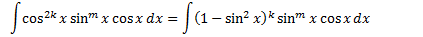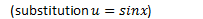This is a polynomial. Expand then integrate.

1.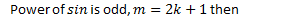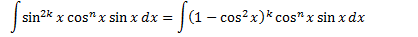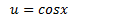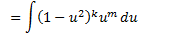1.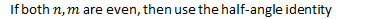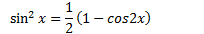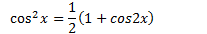Similarly, we can evaluate the integral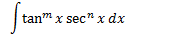Created by Tim Pei with Microsoft OneNote 2010
One place for all your notes and information